Intro to Counting in Groups (Arrays) Math Videos for Kids - Grades K-2
1%
It was processed successfully!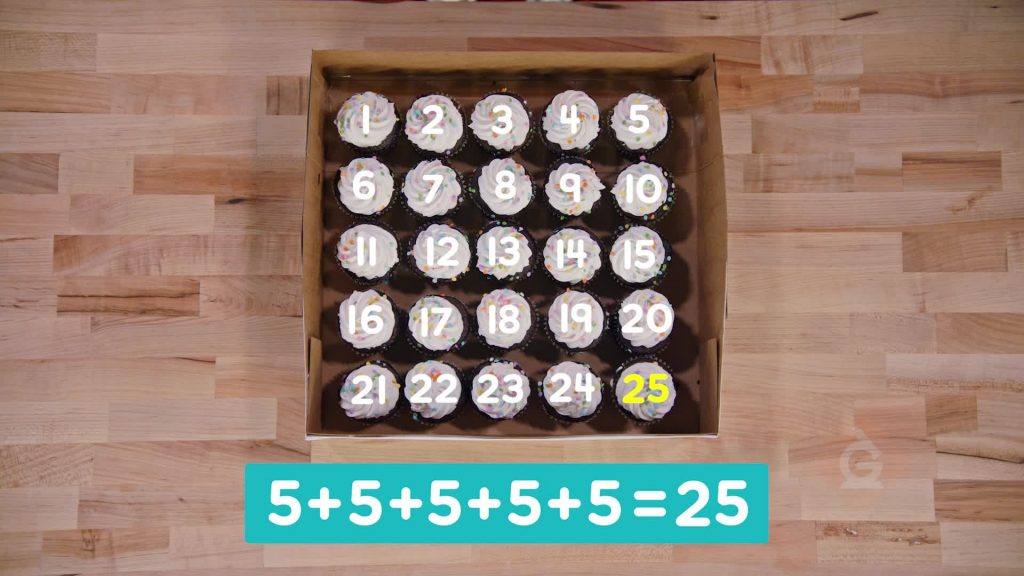WHAT ARE ARRAYS?

Arrays are a way to arrange objects into equal rows. Making arrays with objects makes them easier to count. You can skip count, or repeatedly add, to find how many objects are in an array.

To better understand counting in groups (arrays)…

WHAT ARE ARRAYS?. Arrays are a way to arrange objects into equal rows. Making arrays with objects makes them easier to count. You can skip count, or repeatedly add, to find how many objects are in an array. To better understand counting in groups (arrays)…

## LET’S BREAK IT DOWN!

### Line up tennis balls in an array.You have 4 packs of 3 tennis balls. Line up the equal groups. Each group is called a row. Rows go left and right. When you line up equal rows, the whole thing is called an array.

Line up tennis balls in an array. You have 4 packs of 3 tennis balls. Line up the equal groups. Each group is called a row. Rows go left and right. When you line up equal rows, the whole thing is called an array.

### Count googly eyes in groups of 2.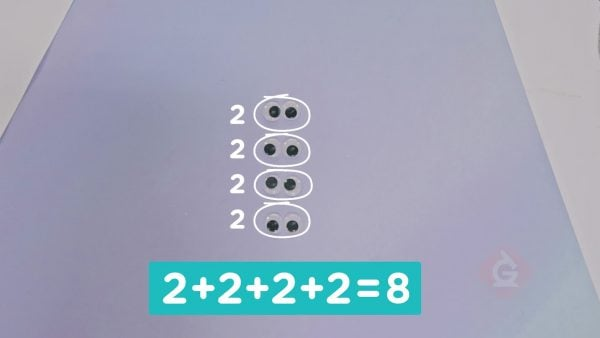You have some googly eyes. Arrange the googly eyes into an array. There are 4 rows with 2 eyes in each row. Count by 2s: 2, 4, 6, 8 eyes. This is the same as 2 + 2 + 2 + 2. Adding equal groups repeatedly is called repeated addition.

Count googly eyes in groups of 2. You have some googly eyes. Arrange the googly eyes into an array. There are 4 rows with 2 eyes in each row. Count by 2s: 2, 4, 6, 8 eyes. This is the same as 2 + 2 + 2 + 2. Adding equal groups repeatedly is called repeated addition.

### Count tennis balls in groups of 3.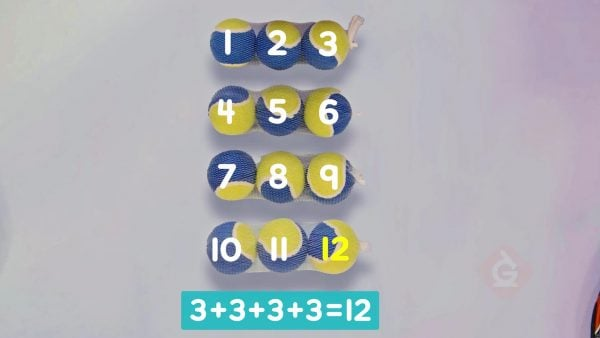Count the tennis balls in the array. There are 4 rows. Each row has 3 balls. Count by 3s four times: 3, 6, 9, 12. There are 12 tennis balls. 3 + 3 + 3 + 3 = 12.

Count tennis balls in groups of 3. Count the tennis balls in the array. There are 4 rows. Each row has 3 balls. Count by 3s four times: 3, 6, 9, 12. There are 12 tennis balls. 3 + 3 + 3 + 3 = 12.

### Count juice boxes in groups of 5.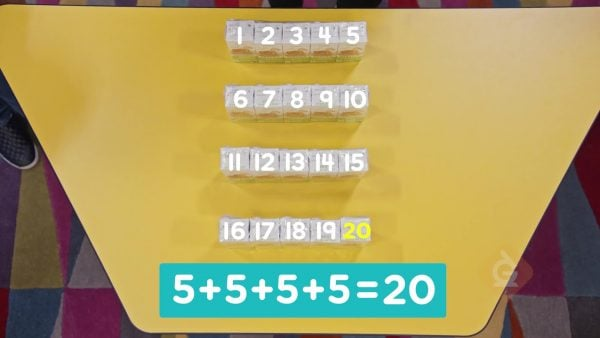You have 4 packs of 5 juice boxes. Line up the juice boxes in 4 equal rows of 5. This is an array. Count by 5s: 5, 10, 15, 20. There are 20 juice boxes. 5 + 5 + 5 + 5 = 20.

Count juice boxes in groups of 5. You have 4 packs of 5 juice boxes. Line up the juice boxes in 4 equal rows of 5. This is an array. Count by 5s: 5, 10, 15, 20. There are 20 juice boxes. 5 + 5 + 5 + 5 = 20.

### Count cupcakes in groups of 5.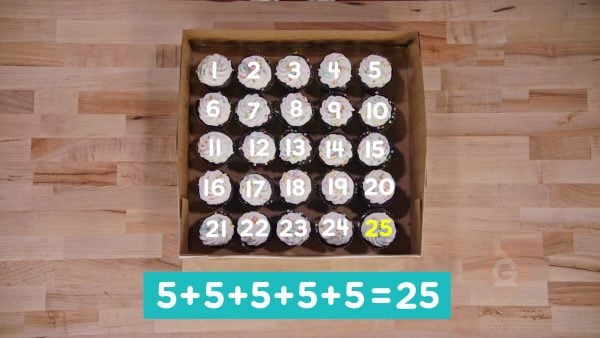You have a box of cupcakes. The cupcakes are in an array. There are 5 rows, and each row has 5 cupcakes. Count by 5s to count them all: 5, 10, 15, 20, 25. You can show this as repeated addition: 5 + 5 + 5 + 5 + 5 = 25.

Count cupcakes in groups of 5. You have a box of cupcakes. The cupcakes are in an array. There are 5 rows, and each row has 5 cupcakes. Count by 5s to count them all: 5, 10, 15, 20, 25. You can show this as repeated addition: 5 + 5 + 5 + 5 + 5 = 25.

## INTRO TO COUNTING IN GROUPS (ARRAYS) VOCABULARY

Skip counting
Counting on by amounts greater than 1.
Equal Groups
Groups with the same number of items.
Putting parts together to find a new total.
Adding equal groups repeatedly, which means adding the same number again and again.
Row
A line of items. Rows go left and right.
Column
A line of items. Columns go up and down.
Items lined up in equal rows.

## INTRO TO COUNTING IN GROUPS (ARRAYS) DISCUSSION QUESTIONS

Repeated addition is adding the same number over and over again. For example, 3 + 3 + 3 + 3 is repeated addition.

### What is a row?

A row is a group of objects in a line. Rows go left and right.

### What is an array?

An array is a group of equal rows that are lined up.

### [Draw an array with 3 rows of 4 dots each on the board.] How many dots are in each row? How can you use repeated addition to find the total number of dots?

There are 4 dots in each row. Add 4 + 4 + 4 = 12.

Add 5 + 5 = 10.
X

## Success

We’ve sent you an email with instructions how to reset your password.
Ok